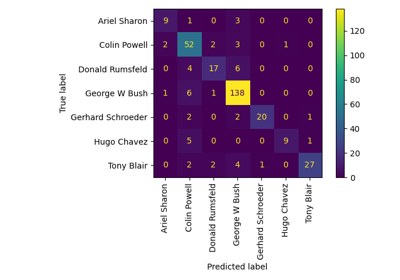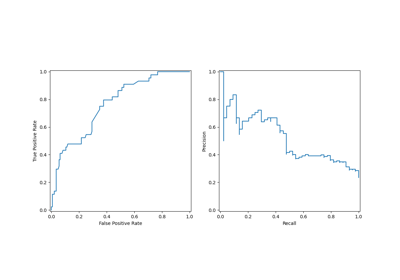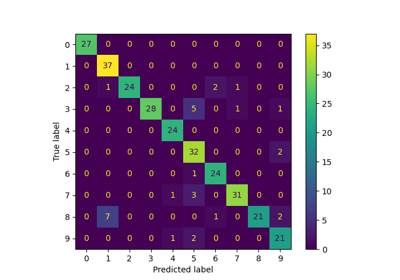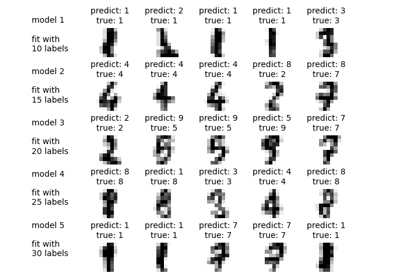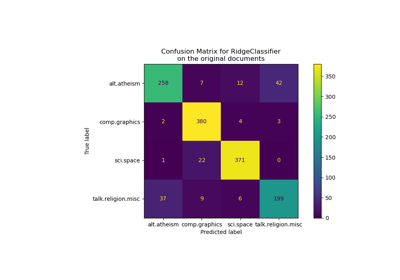# sklearn.metrics.confusion_matrix¶

sklearn.metrics.confusion_matrix(y_true, y_pred, *, labels=None, sample_weight=None, normalize=None)[source]

Compute confusion matrix to evaluate the accuracy of a classification.

By definition a confusion matrix $$C$$ is such that $$C_{i, j}$$ is equal to the number of observations known to be in group $$i$$ and predicted to be in group $$j$$.

Thus in binary classification, the count of true negatives is $$C_{0,0}$$, false negatives is $$C_{1,0}$$, true positives is $$C_{1,1}$$ and false positives is $$C_{0,1}$$.

Read more in the User Guide.

Parameters
y_truearray-like of shape (n_samples,)

Ground truth (correct) target values.

y_predarray-like of shape (n_samples,)

Estimated targets as returned by a classifier.

labelsarray-like of shape (n_classes), default=None

List of labels to index the matrix. This may be used to reorder or select a subset of labels. If None is given, those that appear at least once in y_true or y_pred are used in sorted order.

sample_weightarray-like of shape (n_samples,), default=None

Sample weights.

New in version 0.18.

normalize{‘true’, ‘pred’, ‘all’}, default=None

Normalizes confusion matrix over the true (rows), predicted (columns) conditions or all the population. If None, confusion matrix will not be normalized.

Returns
Cndarray of shape (n_classes, n_classes)

Confusion matrix whose i-th row and j-th column entry indicates the number of samples with true label being i-th class and prediced label being j-th class.

References

1

Wikipedia entry for the Confusion matrix (Wikipedia and other references may use a different convention for axes)

Examples

>>> from sklearn.metrics import confusion_matrix
>>> y_true = [2, 0, 2, 2, 0, 1]
>>> y_pred = [0, 0, 2, 2, 0, 2]
>>> confusion_matrix(y_true, y_pred)
array([[2, 0, 0],
[0, 0, 1],
[1, 0, 2]])

>>> y_true = ["cat", "ant", "cat", "cat", "ant", "bird"]
>>> y_pred = ["ant", "ant", "cat", "cat", "ant", "cat"]
>>> confusion_matrix(y_true, y_pred, labels=["ant", "bird", "cat"])
array([[2, 0, 0],
[0, 0, 1],
[1, 0, 2]])


In the binary case, we can extract true positives, etc as follows:

>>> tn, fp, fn, tp = confusion_matrix([0, 1, 0, 1], [1, 1, 1, 0]).ravel()
>>> (tn, fp, fn, tp)
(0, 2, 1, 1)


## Examples using sklearn.metrics.confusion_matrix¶